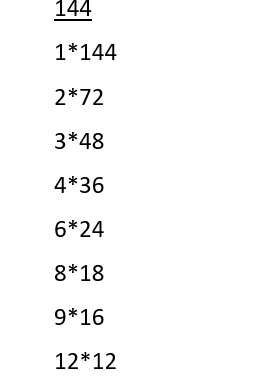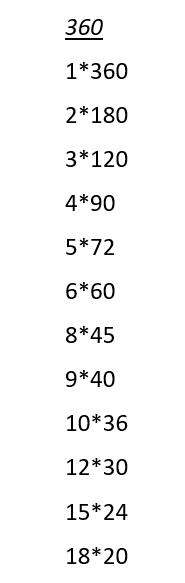# TurtleStitch

### How many times do I want to repeat?

When you are trying to work out the number of repetitions needed to complete a shape, you can use the highest common factor (HCF) method.

I will be using the example of a star. To start off with, you know that you need a move 10 steps and turn degrees button. The move steps can be 10 or 100, it depends on how big you want the shape to be. In this case, the move steps will be set to 100 and the degrees will be set to 144.

Now, to figure out how many times to repeat this block of code, we are going to use the highest common factor method.

You need to get the HCF of the turn degrees and 360 degrees as you are going right around. To start off, find all the multiples of 144 and 360, you can use a calculator or pen to paper for this.Now, find the multiples of 360:Find the HCF of both. In this case, it is 72.

To get your final repetition number, divide 360 by 72, your highest common factor. This equals 5. When you put this block of code into your project on TurtleStitch, you should get a full star. This is how you get the number of repetitions needed to complete your shape.

Created by: Hope Boyle